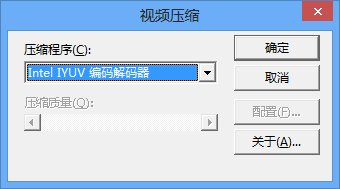### 一、写入图像

#### 程序

```#include <opencv2\highgui\highgui.hpp>
#include <iostream>

using namespace cv;
using namespace std;

int main(int argc, char **argv)
{
Mat img(650, 600, CV_16UC3, Scalar(0, 50000, 50000));
if (img.empty())
{
cout << "加载图像失败！" << endl;
system("pause");
return -1;
}

vector<int> compression_params;
compression_params.push_back(CV_IMWRITE_JPEG_QUALITY);
compression_params.push_back(98);

bool bSuccess = imwrite("C:\\Users\\mingceng\\Documents\\TestImage.jpg", img, compression_params);
if (!bSuccess)
{
cout << "保存图像失败！" << endl;
}

namedWindow("MyWindow", CV_WINDOW_AUTOSIZE);
imshow("MyWindow", img);

waitKey(0);

destroyWindow("MyWindow");

return 0;
}```

#### 解释

`bool imwrite( const string& filename, InputArray img, const vector<int>& params=vector<int>())`

• filename - 指定目标文件的路径和名称
• img -  要保存的图像
• params - 这是指定图像格式的int向量
• JPEG 格式 - 首先 push_back CV_IMWRITE_JPEG_QUALITY 然后是一个0-100的数（数越大质量越好）。如果想获取最佳质量的输出，就使用100，但是值越大，写入图像需要的时间越长
• PNG 格式 - 首先puch_back CV_IMWRITE_PNG_COMPRESSION然后是一个0-9的数(值越大压缩越好，也更慢)

### 二、写入视频

#### 程序

```#include <opencv2\highgui\highgui.hpp>
#include <iostream>

using namespace cv;
using namespace std;

int main(int argc, char **argv)
{
VideoCapture cap(0);
if (!cap.isOpened())
{
cout << "不能打开摄像头设备！" << endl;
return -1;
}

namedWindow("MyVideo", CV_WINDOW_AUTOSIZE);
double dWidth = cap.get(CV_CAP_PROP_FRAME_WIDTH);
double dHeight = cap.get(CV_CAP_PROP_FRAME_HEIGHT);

cout << "帧尺寸：" << dWidth << "x" << dHeight << endl;

Size frameSize(static_cast<int>(dWidth), static_cast<int>(dHeight));

VideoWriter oVideoWriter("C:\\Users\\mingceng\\Documents\\MyVideo.avi", CV_FOURCC('D', 'I', 'V', 'X'), 10, frameSize, true);

if (!oVideoWriter.isOpened())
{
cout << "初始化VideoWriter失败！" << endl;
return -1;
}

while (1)
{
Mat frame;
if (!bSuccess)
{
cout << "不能从视频中读取帧！" << endl;
break;
}
oVideoWriter.write(frame);
imshow("MyVideo", frame);
if (waitKey(10) == 27)
{
cout << "按下ESC键！" << endl;
break;
}

}

return 0;
}```

#### 解释

`Size frameSize(static_cast<int>(dWidth), static_cast<int>(dHeight))`

`VideoWriter::VideoWriter(const string& filename, int fourcc, double fps, Size frameSize, bool isColor=true)`

• const string& filename - 指定输出文件的路径和名称
• int fourcc - 指定用于压缩视频的编解码器的四字符代码。你的电脑可能不支持一些编解码器，所以如果保存视频失败，尝试另外的编解码器，下面是一些常见的编解码器：
• CV_FOURCC('D', 'I', 'V', '3') ——DivX MPEG-4
• CV_FOURCC('M', 'P', '4', '2') —— MPEG-4
• CV_FOURCC('D', 'I', 'V', 'X') —— DivX
• CV_FOURCC('P','I','M','1') —— MPEG-1
• CV_FOURCC('I', '2', '6', '3') —— ITU H.263
• CV_FOURCC('M', 'P', 'E', 'G') —— MPEG-1

完整的列表可以在这里找到。
对于windows用户，可以使用CV_FOURCC_PROMPT（-1）弹出一个对话框选择压缩方法和额外的压缩参数。• double fps - 视频流的帧速率。我在程序里使用10，可以尝试其他值，但应该是编解码器支持的fps，所以使用一个合适的值。
• Size frameSize - 指定视频流每帧的宽和高的Size对象。
• bool isColor - 如果想保存一个彩色视频，这个值为true，否则为false。
` if ( !oVideoWriter.isOpened() )`

`void write(const Mat& image)`